Identifying fractions
Identifying fractions on a number line
Compare and Order Fractions
With Common Denominators
Subtracting Fractions With Common Denominators
Equivalent Fractions
Fractions & Vocab
Fraction Number Stories
100

What fraction of the star is shaded?1/2

100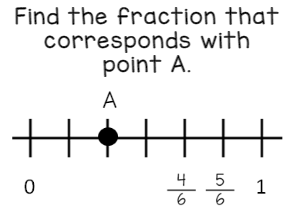2/6

100

Compare the fractions using <, >, or =

5/6     4/6

5/6  >  4/6

100

Add the fractions:   2/5  +  3/5

1 OR 5/5

100

Subtract the fractions:   4/7 - 1/7

3/7

100

Write two equivalent fractions for 2/3

WHAT IS 4/6 6/9 8/12 10/15

100

What is the top number of a fraction called?

Numerator

100

Five people share 10 dimes. What fraction of dimes does each person get?

2/10

200

What fraction of the rectangle is shaded pink?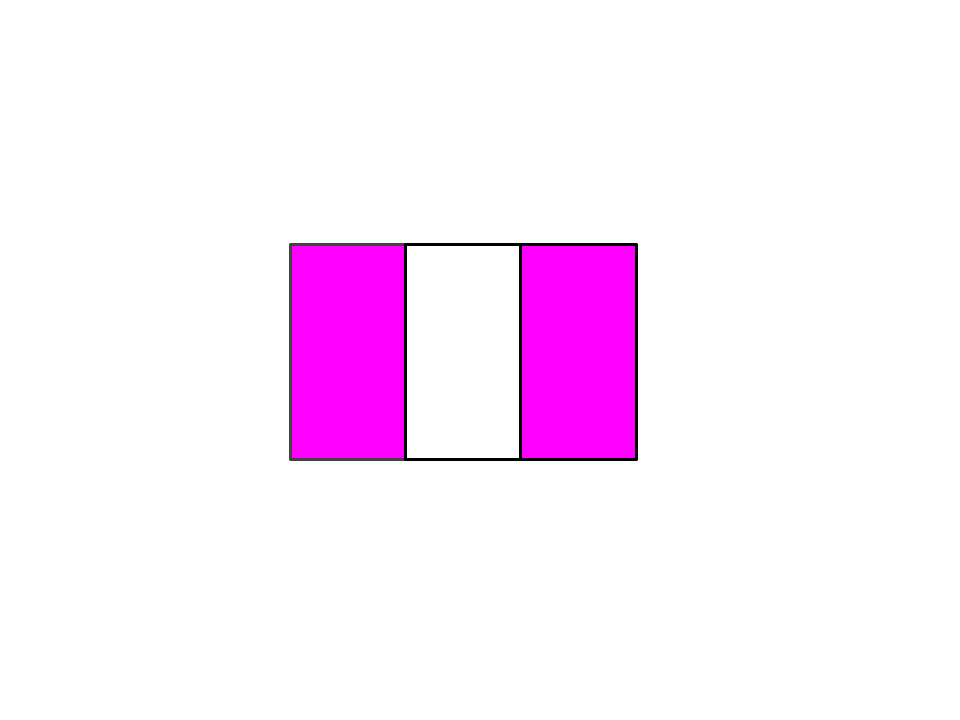2/3

200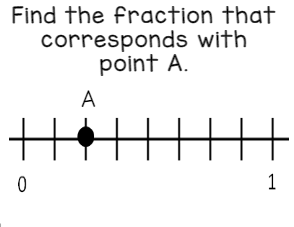2/8

200

Compare the fractions using <, >, or =.

3/4     3/8

3/4  >  3/5

200

11/20

200

Subtract the fractions:  19/20  -  6/20

13/20

200

Write two equivalent fractions for 1/3.

2/6,  3/9,  4/12,  5/15

200

What are the total parts of the whole in a fraction called?

Denominator

200

Jason had 25 quarters. He put 7 of them in his bank. What fraction of quarters did Jason put in his bank?

7/25

300

What fraction of the shape is red?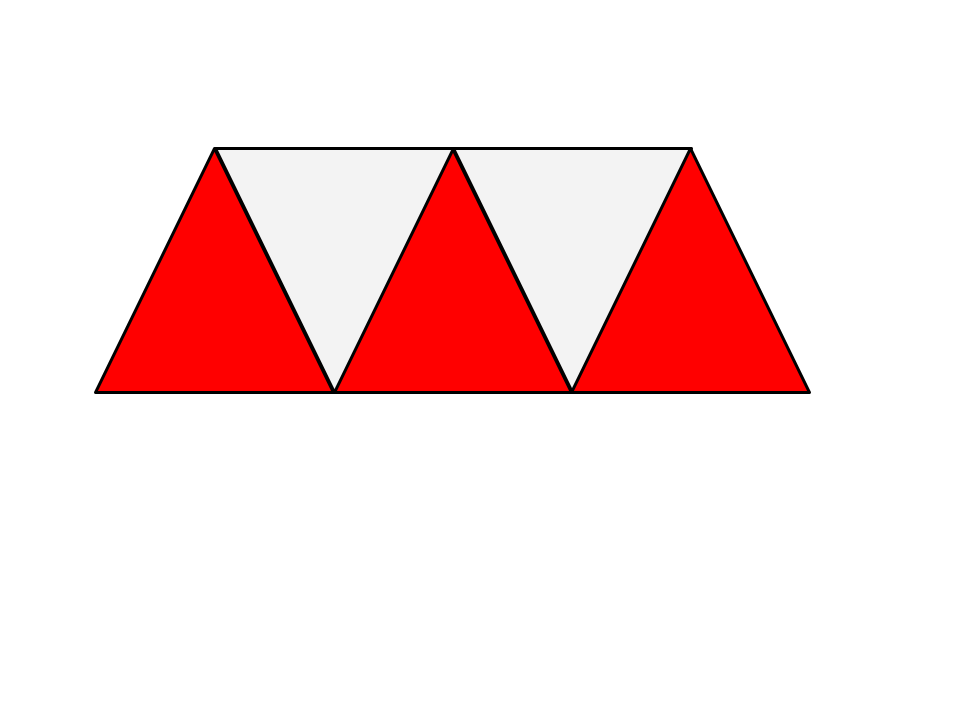3/5

300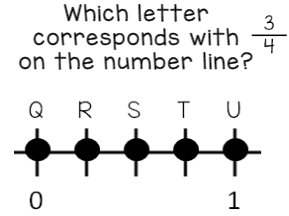T

300

Compare the fractions using <, >, or =.

10/20   10/12

10/20 < 10/12

300

4/8 or 1/2

300

Subtract the fractions:  20/14 -  8/14

12/14

300

Write two equivalent fractions for 5/10.

1/2,  10/20,  15/30,  20/40

300

What does the fraction bar in the middle of a fraction mean?

Out of or divided by

300

Max ate 1/6 of the pizza. How much of the pizza is left?

5/6

400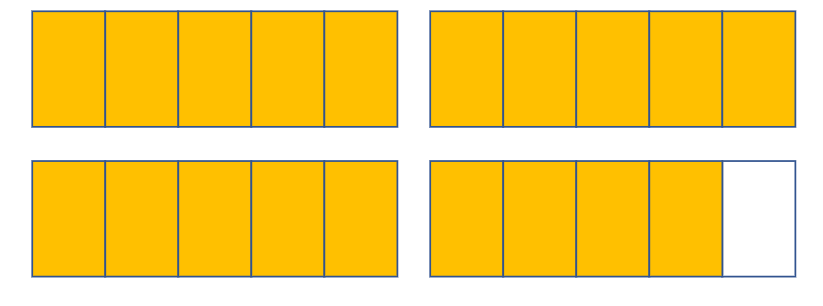3 4/5

400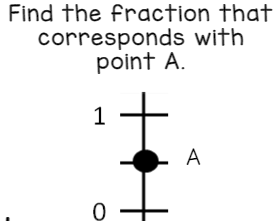1/2

400

Compare the fractions using <, >, or =.

1/2   5/10

1/2 = 5/10
400

65/100

400

8/9 -  6/9

2/9

400

Write two equivalent fractions for 3/4.

6/8,  9/12,  15/20,  18/24

400

What vocabulary word means the same as equal?

Equivalent

400

Sarah painted 1/2 of the wall. Jake painted 1/4 of the wall. Anna painted 1/8 of the wall. Who painted the largest section of the wall?

Sarah

500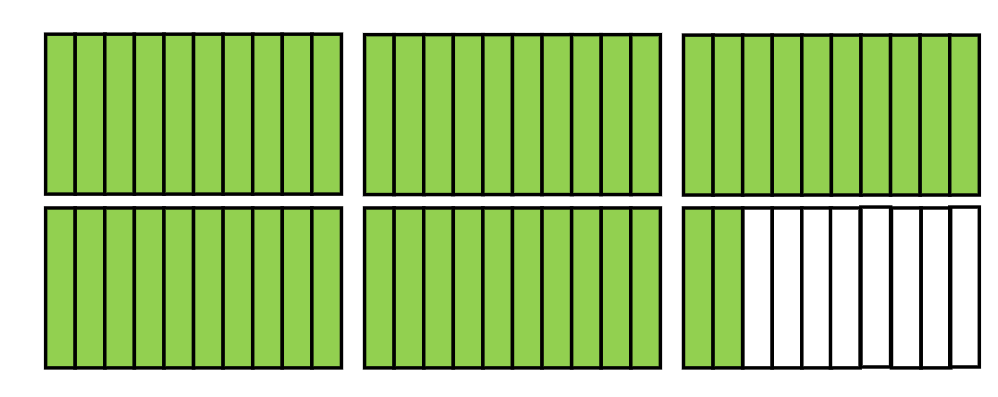5 2/10

500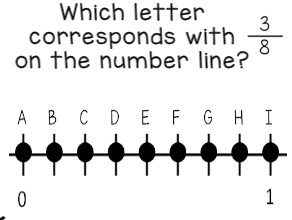D

500

Compare the fractions using >, <, =

2/10     20/100

2/10 = 20/100

500

3 1/5 + 2 2/5 =

28/5 or 5 3/5

500

1/4 -  2/4 =

3/4

500

Write two equivalent fractions for 1.

1/1,  2/2,  3/3,  4/4

500

Name a way fractions can be used in real life? Think, why am I learning fractions?

to divide food, money or other items

500

What fraction of an hour is 15 minutes?1/4

Click to zoom